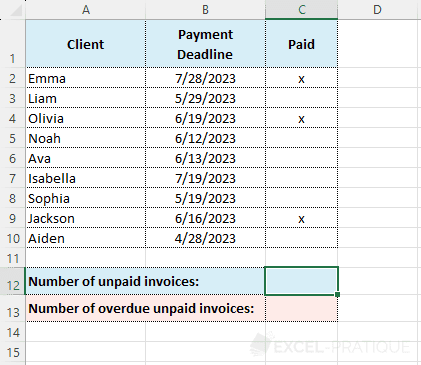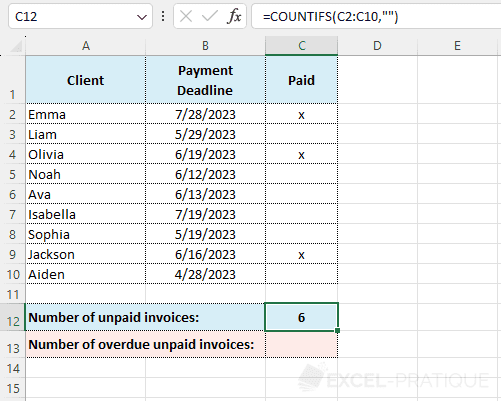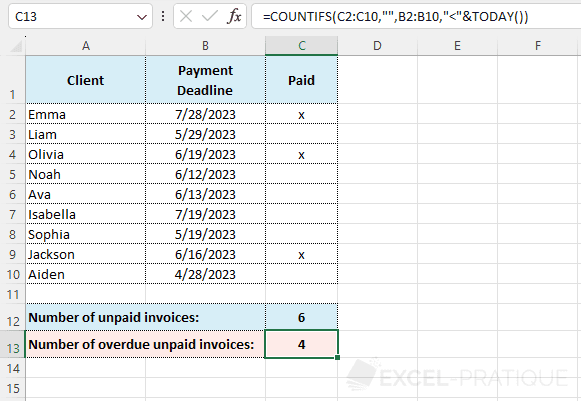# Excel Function: COUNTIFS

The COUNTIFS function applies criteria to cells across multiple ranges and counts the number of times all criteria are met (the COUNTIF function is limited to a single criteria).

Usage:

`=COUNTIFS(Criteria_range1, Criteria1)`

or

`=COUNTIFS(Criteria_range1, Criteria1, Criteria_range2, Criteria2, ...)`

## Example of use

The goal here is to first calculate the number of unpaid invoices (= 1 criteria) and then the number of overdue unpaid invoices (= 2 criteria):Enter into the COUNTIFS function:

• Criteria_range1: the range to be evaluated with Criteria1 (here, the "Paid" column)
• Criteria1: the evaluation criteria (here, `""` to check if the cell is empty)

The formula is therefore here:

``=COUNTIFS(C2:C10,"")``Since there is only one criteria, the COUNTIF function could have also been used here.

To then get the number of overdue unpaid invoices, a second criteria must be added to the formula which will check if the payment deadline has passed (i.e., a date smaller than TODAY):

``=COUNTIFS(C2:C10,"",B2:B10,"<"&TODAY())``